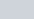Find the sum of n terms of an A.P. whose nth terms is given by an = 5 − 6n.
Question:

Find the sum of n terms of an A.P. whose nth terms is given by an = 5 − 6n.

Solution:

Here, we are given an A.P., whose $n^{\text {th }}$ term is given by the following expression, $a_{n}=5-6 n$

So, here we can find the sum of the $n$ terms of the given A.P., using the formula, $S_{n}=\left(\frac{n}{2}\right)(a+l)$

Where, a = the first term

l = the last term

So, for the given A.P,

The first term (a) will be calculated usingin the given equation for nth term of A.P.

$a=5-6(1)$

$=5-6$

$=-1$

Now, the last term (l) or the nth term is given

$a_{e}=5-6 n$

So, on substituting the values in the formula for the sum of n terms of an A.P., we get,

$S_{n}=\left(\frac{n}{2}\right)[(-1)+5-6 n]$

$=\left(\frac{n}{2}\right)[4-6 n]$

$=\left(\frac{n}{2}\right)(2)[2-3 n]$

$=(n)(2-3 n)$

Therefore, the sum of the $n$ terms of the given A.P. is $(n)(2-3 n)$.